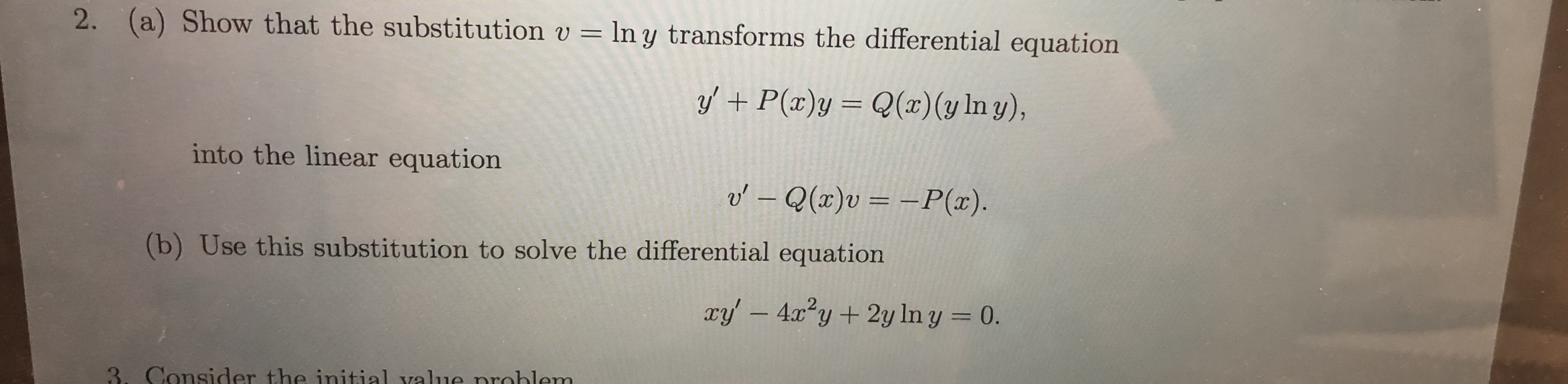2. (a) Show that the substitution vln y transforms the differential equationQ(x) (y In y),yP(x)yinto the linear equationo'- Q(x)v = -P(x)(b) Use this substitution to solve the differential equationry' -4xy2y In y = 0.3. Consider the initial value nroblem

Questionhelp_outlineImage Transcriptionclose2. (a) Show that the substitution v ln y transforms the differential equation Q(x) (y In y), yP(x)y into the linear equation o'- Q(x)v = -P(x) (b) Use this substitution to solve the differential equation ry' -4xy2y In y = 0. 3. Consider the initial value nroblem fullscreen
Step 1

To transform the given differential equation using the appropriate substitution, and hence solve the simpler equation

Step 2

(a) Effect of substitution on the derivatives of y and v.

Step 3

(a) verifi...

Want to see the full answer?

See Solution

Want to see this answer and more?

Our solutions are written by experts, many with advanced degrees, and available 24/7

See Solution
Tagged in

Math